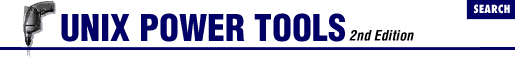#Chapter 47C Shell Programming...NOT## 47.4 C Shell Variable Operators and Expressions

### 47.4.1 Variables

In the following substitutions, braces (`{}`) are optional, except when needed to separate a variable name from following characters that would otherwise be a part of it. The array argv (the command-line arguments) is used as an example, but any csh array name may be used.
 \${`var`} The value of variable var. \${`var`[`i`]} Select word or words in position i of var. i can be a single number, a range `m``-``n`, a range `-``n` (missing `m` implies 1), a range `m``-` (missing `n` implies all remaining words), or `*` (select all words). i can also be a variable that expands to one of these values. \${#`var`} The number of words in var. \${#argv} The number of command-line arguments. \${argv[`n`]} Individual arguments on command line (positional parameters). n is a number (1, 12, etc.). \${`n`} Same as `\${argv[``n``]}`. \${argv[*]} All arguments on command line. \$* Same as `\$argv[*]`. \${argv[\$#argv]} The last argument. \${?`var`} Return 1 if var is set; 0 if var is not set. ! \${?`var`} Return 0 if var is set; 1 if var is not set. \$\$ Process number of current shell; useful as part of a filename for creating temporary files with unique names. \$< Read a line from standard input.

### 47.4.2 Expressions

Expressions are used in C shell `@`, if, and while statements to perform arithmetic, string comparisons, file testing, and so on. exit and set can also specify expressions. Expressions are formed by combining variables and constants with operators that resemble those in the C programming language. Operator precedence is the same as in C but can be remembered as follows:

1. `* / %`

2. `+ -`

Group all other expressions inside ( ). Parentheses are required if the expression contains `<`, `>`, `&`, or `|`.

### 47.4.3 Operators

Operators can be one of the following types:

#### 47.4.3.1 Assignment Operators

 = Assign value. += -= Reassign after addition/subtraction. *= /= %= Reassign after multiplication/division/remainder. &= ^= |= Reassign after bitwise AND/XOR/OR. ++ Increment. - Decrement.

#### 47.4.3.2 Arithmetic Operators

 * / % Multiplication; integer division; modulus (remainder). c+ - Addition; subtraction.

#### 47.4.3.3 Bitwise and Logical Operators

 ~ Binary inversion (one's complement). ! Logical negation. << >> Bitwise left shift; bitwise right shift. & Bitwise AND. ^ Bitwise exclusive OR. | Bitwise OR. && Logical AND. || Logical OR. { `cmd` } Return 1 if command cmd is successful; 0 otherwise. Note that this is the opposite of cmd's normal return code. The status variable may be more practical.

#### 47.4.3.4 Comparison Operators

 == != Equality; inequality. <= >= Less than or equal to; greater than or equal to. < > Less than; greater than. =~ String on left matches a filename pattern on the right containing `*`, `?`, or `[`...`]`. !~ String on left does not match a filename pattern containing `*`, `?`, or `[`...`]`.

#### 47.4.3.5 File Inquiry Operators

Command substitution and filename expansion are performed on file before the test is performed.
 -d `file` The file is a directory. -e `file` The file exists. -f `file` The file is a plain file. -o `file` The user owns the file. -r `file` The user has read permission. -w `file` The user has write permission. -x `file` The user has execute permission. -z `file` The file has zero size. ! Reverse the sense of any of the above inquiries.

### 47.4.4 Examples

The following examples show `@` commands and assume n = 4:
ExpressionValue of \$x
@ x = (\$n > 10 || \$n < 5)1
@ x = (\$n >= 0 && \$n < 3)0
@ x = (\$n << 2)16
@ x = (\$n >> 2)1
@ x = \$n % 20
@ x = \$n % 31

The following examples show the first line of if or while statements:

Expression

Meaning

while (\$#argv != 0)

While there are command-line (argv) arguments ...

if (\$today == Fri)

If the first word is Fri...

if (\$file !~ *.[zZ])

If the file doesn't end with .z or .Z ...

if (\$argv =~ chap?)

If the first argument is chap followed by a single character...

if (-f \$argv)

If the first argument is a plain file...

if (! -d \$tmpdir)

If tmpdir is not a directory...

- DG from O'Reilly & Associates' UNIX in a Nutshell (SVR4/Solaris)47.3 Conditional Statements with if47.5 Using C Shell Arrays